# Isolated charge particles in electric and magnetic fields¶

This chapter deals with charge particle motion.

Particles experience three different types of interactions

1. collisions (direct or indirect): due to direct interaction between particles.
2. grativity: when particles have mass
3. electromagnetic forces: when particles have charge

In this chapter, we ignore collisions. They will be treated in a different chapter.

## Force on a particle caused by gravity¶

Gravitation is always present in any experiment in a laboratory or in astrophysical settings. However, it can be ignored when charged particles are surrounded by large electric fields or magnetic fields. However, one should always estimate the impact of any force to assess which are negligible and which is not. Ultimately, developing a model where all forces are taken into account will lead to unwanted complexity.

Overall, the best approach is back of the envelope calculation before taking on big computational problems and apply the full force of numerical analysis to generate large 3-dimensional datasets.

### Gravitational fields with small spatial variations¶

When the change in gravitation is small compared to the particle trajectory, the force applied on a particle of mass $m$ is simply its weight. $$\vec{F}=m\vec{g}\tag{2.1}$$ We now need to define the trajectory as a function of time using Newton's second law $$\frac{d\vec{p}}{dt}=\sum_i{\vec{F_i}}$$ $\vec{p}$ is the particle momentum given by $\vec{p}=m\vec{v}$ using the particle mass and it velocity $\vec{v}$. Clearly, we have only one force here! So, we shall simplify it right away to $$\frac{d\vec{p}}{dt}=m\vec{g}\tag{2.2}$$ It is possible to integrate Eq. $(2.2)$ using a very simple integration method for Ordinary Differential Equations.

### Integrating first order ordinary differential equations¶

Newton's second law, i.e. Eq. $(2.1)$, can be discretized. Discretization means in this context that $dt$, representing an infinitely small amount of time, is turned into $\Delta t$, a finite amount of time. We can rewrite Newton's law as $$\frac{\Delta p}{\Delta t}=\vec{F}\tag{2.3}.$$ Clearly, we get from this $p_{t+\Delta t}-p_t=\vec{F}\Delta t$. If we know the momentum and the force at $t$, then we can compute the new momentum at $\Delta t$. To extract the trajectory from this equation, we have to realize that $\vec{v}=\frac{d\vec{r}}{dt}$ where $\vec{r}(t)=(x(t),y(t),z(t))$, the coordinate of the particle at the time $t$. We can rewrite Eq. $(2.3)$ as $$m\vec{v}_{t+\Delta t}-m\vec{v}_t=m\vec{g}\Delta t$$ or $$\vec{v}_{t+\Delta t}-\vec v_t=\vec g\Delta t$$ It seems that this trajectory does not dependent on the particle mass! This is a remarkable result. An electron inside a gravitational field falls as fast as a proton, which is 2000 times more massive. We need to expand this equation to our coordinate system $(x,y,z)$

Here is the system of equations we need to solve for. $$v_{x,t+\Delta t}=g_x\Delta t+v_{x,t}\\ v_{y,t+\Delta t}=g_y\Delta t+v_{y,t}\\ v_{z,t+\Delta t}=g_z\Delta t+v_{z,t}$$ However, this system is not giving the trajectory $(x(t),y(t),z(t))$ but the velocity $(v_x(t),v_y(t),v_z(t))$. Fortunately, we know that $\vec{v}=\frac{d\vec{r}}{dt}$.

Now we find the trajectory using $$x_{t+\Delta t}=v_{x,t+\Delta t}\Delta t+x_t\\ y_{t+\Delta t}=v_{y,t+\Delta t}\Delta t+y_t\\ z_{t+\Delta t}=v_{z,t+\Delta t}\Delta t+z_t$$ We are now ready to do some computations using python.

## Using python to solve the free fall of an electron inside Earth's gravity¶

We are going to solve equation $(2.1)$ using the following conditions. First, $$\vec{g}=(0,g_y,0)$$ with $g_y=-9.81m/s^2$. Our initial conditions are $$v_x=0\\v_y=0\\v_z=0$$ and $$x=0\\y=0\\z=0$$ Clearly, nothing happens in the z direction. So, we will ignore it for now.

Let's start by importing our tools into the python code, math, numpy and matplotlib.

In :
import math
from math import sqrt as sqrt
import numpy as np
import matplotlib.pyplot as plt
#to display inside the notebook!
%matplotlib inline


Then we define our main (i.e. global) variables, all in mks

In :
#initial time
t_initial=0
#final time
t_final=10
#time step
dt=0.5
#number of time steps declared as integer
n = int((t_final-t_initial)/dt)
#electron mass
m=9.10938356e-31
#gravity at sea level
gx=0
gy=-9.81
#initial velocity
vx_initial=0
vy_initial=0
#we use the momemtum to be physically sound, even if using velocity is more computationally effecient
px_initial=m*vx_initial
py_initial=m*vy_initial
#initial position
x_initial=0
y_initial=0


Now we declare our arrays. While in python we do not have to declare variables like in C or Fortran, arrays are special. Here we just declare at one element using np (i.e. numpy). However, since we know the total number of steps, we could have assigned them right from the start. Avoid using np.empty(...) as this function allocates memory but does not set it to a given value (like 0s).

In :
x=np.zeros(1)
y=np.zeros(1)


We now declare other variables which will be used inside the main part of the code. Technically we do not have to do this, but it makes the code more readable.

In :
px_old=px_initial
py_old=py_initial
x_old=x_initial
y_old=y_initial


We now work on our main loop. It contains the algorithm that will solve our ordinary differential equation. We start with the for loop. for i in range(1,n,1): This loop goes from 1 to n with a step of 1. Note that there is a column : at the end that indicates to python that the code included in the loop is below. Indentations are used to tell python which piece of code is in the loop. So indentations are important in python. To put a piece of code outside of the loop, you need to indent back to the left.

In :
for i in range(1,n,1):
fx=m*gx #this is the force along x
fy=m*gy #this is the force along y
px_new=fx*dt+px_old #new momentum
py_new=fy*dt+py_old
vx=px_new/m #new velocity
vy=py_new/m
x_new=vx*dt+x_old #new position
y_new=vy*dt+y_old
x=np.append(x,[x_new]) #the positions are appended at the end of the x and y arrays.
y=np.append(y,[y_new])
x_old=x_new # we now replace the old data with the new ones
y_old=y_new
px_old=px_new
py_old=py_new
# and the loop starts again.


i is the step counter going from 1 to n, with s step of 1. Note the indent to indicate python that this piece of code is inside the for loop. Note that we note only compute the components of the force $\vec{F}$ fx and fy but both variables are also declared at the same time. This goes for the other variables also. It is time to plot the results now

In :
fig, ax = plt.subplots(1, 1, figsize=(8, 8))
# Now, we draw our points with a gradient of colors.
ax.scatter(x, y, c=range(n), linewidths=0,marker='o', s=15, cmap=plt.cm.jet,)
ax.axis('equal')
ax.set_axis_off()So we have just plotted the electron trajectory inside the Earth gravitational field. Each dot corresponds to the position of the particle at each time steps, the time difference is constant. The increasing distance between each point is sign of acceleration. But we can also interpolate the whole trajectory by adding points in between. These points are used to make the graph more readable. However, since they are not computed with the main algorithm they are only an approximation of the trajectory. Let's create two other arrays that interpolates the initial arrays x and y.

In :
k = 100
x_interpolated = np.interp(np.arange(n * k), np.arange(n) * k, x)
y_interpolated = np.interp(np.arange(n * k), np.arange(n) * k, y)


And see what we get

In :
fig, ax = plt.subplots(1, 1, figsize=(8, 8))
# Now, we draw our points with a gradient of colors.
ax.scatter(x_interpolated, y_interpolated, c=range(n*k), linewidths=0,
marker='o', s=15, cmap=plt.cm.jet,)
ax.axis('equal')
ax.set_axis_off()We can make a simple plotting function by rewriting the code above slightly.

In :
def plot_2D(x,y,n_interpolated,plot_size,plot_axes):
n = len(x)
k = n_interpolated
x_interpolated = np.interp(np.arange(n * k), np.arange(n) * k, x)
y_interpolated = np.interp(np.arange(n * k), np.arange(n) * k, y)
fig, ax = plt.subplots(1, 1, figsize=(plot_size, plot_size))
# Now, we draw our points with a gradient of colors.
ax.scatter(x_interpolated, y_interpolated, c=range(n*k), linewidths=0,
marker='o', s=2*plot_size, cmap=plt.cm.jet,) #s is the size of the plotted symbol 'o'
ax.axis('equal')
if (plot_axes!="axes"):#so we can switch the axis on or off
ax.set_axis_off()
else:
plt.xlabel("x (m)")
plt.ylabel("y (m)")


And we can print easily now.

In :
plot_2D(x_interpolated,y_interpolated,10,4,"no")
plot_2D(x_interpolated,y_interpolated,10,4,"axes")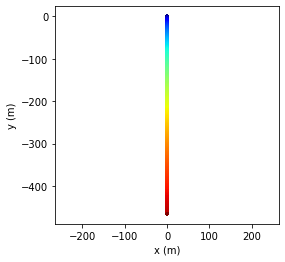## Entr'acte¶

Let's take a break from physics and see if we can use a function to integrate Eq. $(2.3)$. We can reuse the for loop above to generate an ordinary differential equation integrator using $x_0$, $y_0$, $v_{x,0}$, $v_{y,0}$, $t_{initial}$, $t_{final}$ and the number of steps $n_{steps}$ as inputs. We define it as

def OED_integration(x_initial,y_initial,vx_initial,vy_initial,t_initial,t_final,n_steps):


The other variables, like m, t, dt, ... are kept as is and do not have to be part of the input of OED_integration.

In :
def ODE_integration(x_initial,y_initial,vx_initial,vy_initial,t_initial,t_final,n_steps):
x=np.full(1,x_initial) # we do this to initialize the x variable as a numpy array
y=np.full(1,y_initial)
px_old=vx_initial*m
py_old=vy_initial*m
dt=(t_final-t_initial)/n_steps
for i in range(1,n_steps,1):
fx=m*gx #this is the force along x
fy=m*gy #this is the force along y
px_new=fx*dt+px_old #new momentum
py_new=fy*dt+py_old
vx=px_new/m #new velocity
vy=py_new/m
x_new=vx*dt+x[i-1] #new position
y_new=vy*dt+y[i-1] # in python arrays start at index 0
x=np.append(x,x_new) #the positions are appended at the end of the x and y arrays.
y=np.append(y,y_new)
"""The ones below are not needed. We use the ones from the previous step inside the array
x_old=x_new
y_old=y_new"""
px_old=px_new
py_old=py_new
return x,y
# and the loop starts again.


We then create two new arrays and fill them up using ODE_integration(x_initial,y_initial,vx_initial,vy_initial,t_initial,t_final,n_steps)

In :
x,y=ODE_integration(0,0,10,10,0,3,80)
plot_2D(x,y,10,8,"axes")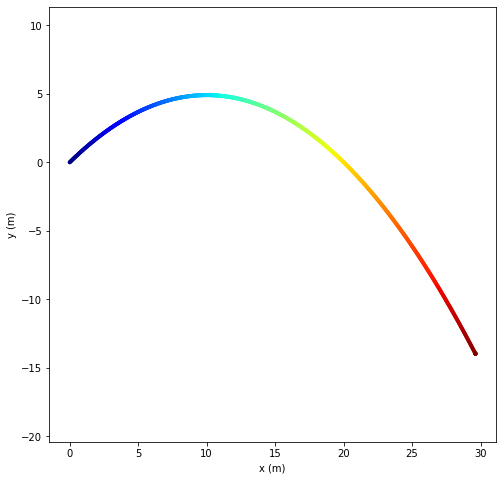## Electrostatic force¶

It is now time to move on to electrostatic forces. A particle with charge $q$ placed inside an electric field $\vec{E}$ is submitted to a force $$\vec F=q\vec E\tag{2.4}$$ Does this formula remind you of another one seen before?

### Physical model¶

Indeed, the particle feels a force that looks like gravity (i.e. Eq. $2.1$). However, there is a subtle difference when we use Newton's second law $$m\frac{d\vec v}{dt}=q\vec E$$ This time around the mass does not go away and we expect the trajectory to depend on the mass. Here is the new system of equations we need to solve for. $$v_{x,t+\Delta t}=\frac{q}{m}E_x\Delta t+v_{x,t}\\ v_{y,t+\Delta t}=\frac{q}{m}E_y\Delta t+v_{y,t}\\ v_{z,t+\Delta t}=\frac{q}{m}E_z\Delta t+v_{z,t}$$ And since $\vec{v}=\frac{d\vec{r}}{dt}$ we get the same system as before for the coordinates, i.e. $$x_{t+\Delta t}=v_{x,t+\Delta t}\Delta t+x_t\\ y_{t+\Delta t}=v_{y,t+\Delta t}\Delta t+y_t\\ z_{t+\Delta t}=v_{z,t+\Delta t}\Delta t+z_t$$

### Using python to solve the free fall of an electron inside an electric field¶

We are going to solve equation $(2.1)$ using the following conditions. First, $$\vec E=(0,E_y,0)$$ with $E_y=-1 V/m$. Our initial conditions are $$v_x=0\\v_y=0\\v_z=0$$ and $$x=0\\y=0\\z=0$$ Clearly, nothing happens in the z direction. So, we will ignore it for now.

Let's redefine our ODE integrator to cope with this new force. We need to initialize the charge of our electron and the electric field in which it is moving, all in MKS.

In :
q=-1.60217662e-19
Ex=0
Ey=1
vx_final=0
vy_final=0
clight=299792458


And then we make our new function, named OED_integration_E.

In :
def ODE_integration_E(x_initial,y_initial,vx_initial,vy_initial,t_initial,t_final,n_steps):
x=np.full(1,x_initial) # we do this to initialize the x variable as a numpy array
y=np.full(1,y_initial)
px_old=vx_initial*m
py_old=vy_initial*m
dt=(t_final-t_initial)/n_steps
for i in range(1,n_steps,1):
fx=q*Ex #this is the force along x
fy=q*Ey #this is the force along y
px_new=fx*dt+px_old #new momentum
py_new=fy*dt+py_old
vx=px_new/m #new velocity
vy=py_new/m
x_new=vx*dt+x[i-1] #new position
y_new=vy*dt+y[i-1] # in python arrays start at index 0
x=np.append(x,x_new) #the positions are appended at the end of the x and y arrays using numpy.
y=np.append(y,y_new)
px_old=px_new
py_old=py_new
print(vx/clight,vy/clight)
print(x[n_steps-1],"m",y[n_steps-1],"m")
return x,y
# and the loop starts again.


Can you guess what changed??

Besides the name (which we changed to avoid overriding the previous function), we changed only two lines

fx=q*Ex #this is the force along x
fy=q*Ey #this is the force along y

We could do this because the previous code conversed physical information and separated force and momentum. While being less efficient computationally, we were able to reuse the code, mostly unchanged.

So let's run it!

In :
x,y=ODE_integration_E(0,0,0,0,0,3,2000)
plot_2D(x,y,1,8,"axes")

0.0 -1759.1576096745716
0.0 m -791073275720.6312 m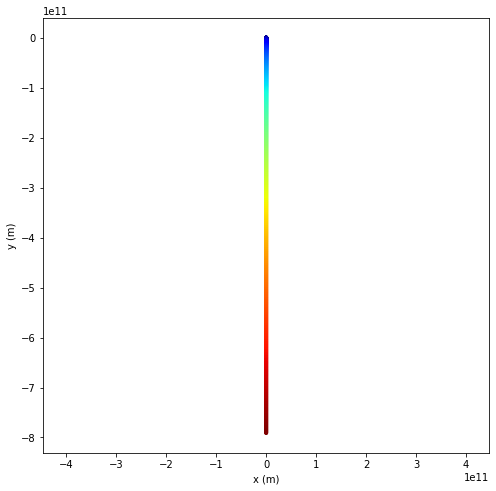That's a long way down. And the velocity is enormous. Did we do something wrong? Let's check it by integrating analytically and make sure the code is correct! $$\frac{d^2y}{dt^2}=\frac{q}{m}E_y\\ \frac{dy}{dt}=\frac{q}{m}E_y t+v_{y0}=\frac{q}{m}E_y t$$ We integrate one last time to get the trajectory $$y=\frac{q}{2m}E_y t^2+y_0=\frac{q}{2m}E_y t^2\tag{2.5}$$ So, for an electron moving inside an electric field of $1V/m$ we get

In :
print(q*Ey/(2.*m)*3.**2,"m")

-791469010225.7589 m


Well! The code gives a very similar answer to the analytical solution. They are not exactly the same because the numerical integration can only be as exact as the analytical solution of Eq. $(2.5)$ for a number of steps that is infinitely large. Of course, we know that the special law of relatively need to be invoked to limit the speed of the electron below the speed of light. So we have integrated our ODE for too long, as the output velocities show. Let's do it for a shorter time, let's say $3\mu s$

In :
x,y=ODE_integration_E(0,0,0,0,0,3e-6,20)
plot_2D(x,y,1,8,"axes")

0.0 -0.0016720357470644369
0.0 m -0.7518955597144708 m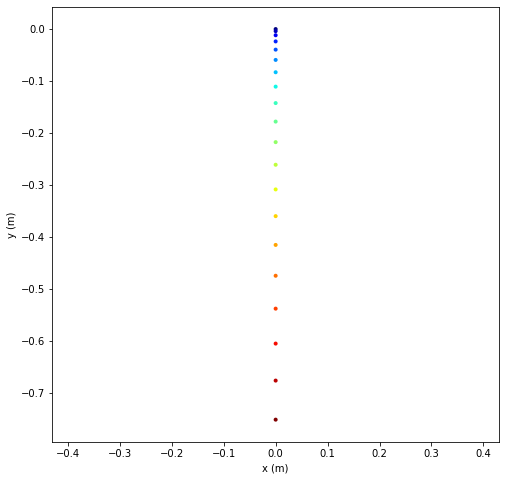Well we are now only at 0.16% the speed of light. So, we can accept that our solution is physically sound. Now let's see if we give an initial velocity of $200km/s$ in both direction

In :
x,y=ODE_integration_E(0,0,2e5,2e5,0,5e-6,60)
plot_2D(x,y,1,8,"axes")

0.0006671281903963041 -0.002217377922960473
0.9833333333333347 m -1.1785496112648044 m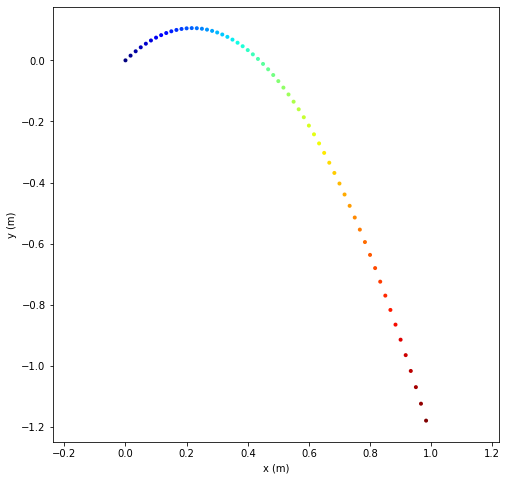While at our geometrical scale, the structure of the gravitational field is straightforward, electric field distributions can be more complex. For instance, the electric field generated by one single particle with charge q is given by $$\vec{E}=\frac{1}{4\pi\varepsilon_0}\frac{q}{r^2}\hat{r}\tag{2.6}.$$ Here $r$ is the distance between the point where the field is measured and the particle location. $\hat{r}$ is the unit vector direction, looking at the measurement location from the charge point of view.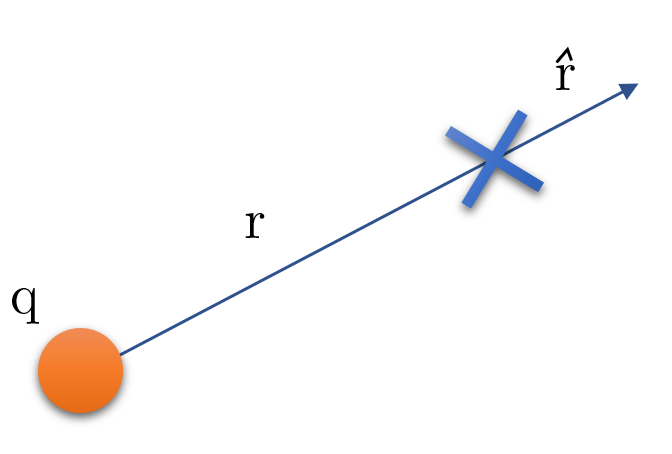Figure 1: The geometry used to compute the electric field generated by a charge q at a remote location (the cross).

If the particle is located at $(x_p,y_p,z_p)$ and we measure the field at the position $(x,y,z)$ then $r$ is simply $$r=\sqrt{(x-x_p)^2+(y-y_p)^2+(z-z_p)^2}$$ and $$\hat{r}=\frac{x-x_p}{r}\hat{x}+\frac{y-y_p}{r}\hat{y}+\frac{z-z_p}{r}\hat{z}.$$ We now take the previous electron and drop it in the electric field created by an immobile proton, with charge $q=+1.6\times10^{-19}C$

In :
def ODE_integration_E_with_charge(charge_location,charge_value,initial_position,initial_velocity,time_frame,n_steps):
loc=np.zeros((3,n_steps)) #index 0 is x, index 1 is y and index 2 is z
loc[:,0]=initial_position
""" Let's start to use vectors and combine notations
the column : is equivalent to writing
loc[0,0]=initial_position
loc[1,0]=initial_position
loc[2,0]=initial_position
"""
v_old=initial_velocity
dt=(time_frame-time_frame)/n_steps
E=[0,0,0]
f=np.zeros(3)
v_new=np.zeros(3)
for i in range(1,n_steps,1):
r=sqrt((loc[0,i-1]-charge_location)**2+(loc[1,i-1]-charge_location)**2+(loc[2,i-1]-charge_location)**2)
if (r<1e-7):
r=1e-7 # we won't divide by 0
r_unit=(loc[:,i-1]-charge_location)/r
E=1./(4*3.1416*8.85e-12)*charge_value/r**2*r_unit
f=q*E
v_new=f/m*dt+v_old #new velocity
loc[:,i]=v_new*dt+loc[:,i-1] #new position
v_old=v_new
return loc


Now let's define a new plotting function that indicates where the motionless charge is located

In :
def plot_2D_with_charge(rc,x,y,n_interpolated,plot_size,plot_axes):
n = len(x)
k = n_interpolated
x_interpolated = np.interp(np.arange(n * k), np.arange(n) * k, x)
y_interpolated = np.interp(np.arange(n * k), np.arange(n) * k, y)
fig, ax = plt.subplots(1, 1, figsize=(plot_size, plot_size))
# Now, we draw our points with a gradient of colors.
ax.scatter(x_interpolated, y_interpolated, c=range(n*k), linewidths=0,
marker='o', s=2*plot_size, cmap=plt.cm.jet,) #s is the size of the plotted symbol 'o'
ax.scatter(rc, rc, c='black', linewidths=0,
marker='x', s=3*plot_size,)
ax.axis('equal')
if (plot_axes!="axes"):#so we can switch the axis on or off
ax.set_axis_off()
else:
plt.xlabel("x (m)")
plt.ylabel("y (m)")

In :
rc=[4e-3,0,0] #charge location
qc=1.6e-19 #charge value
loc=ODE_integration_E_with_charge(rc,qc,(-5e-3,10e-4,0),(500,0,0),(0,50e-6),1000)
plot_2D_with_charge(rc,loc[0,:],loc[1,:],1,7,"axes")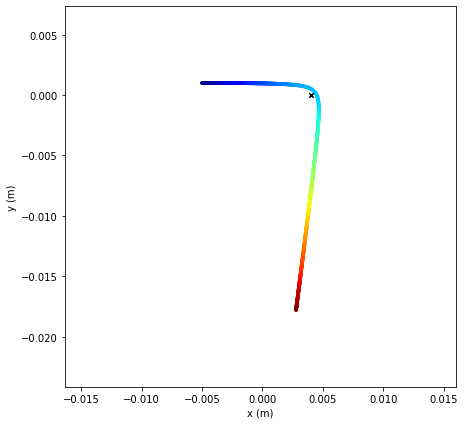## Magnetic force¶

After looking at electrostatic forces, we now study magnetic forces. A particle of charge $q$ and traveling at a velocity $\vec v$ inside a magnetic field $\vec{B}$ is given by $$\vec F=q\vec v\times \vec B$$ In this case, the equation of motion is given by $$m\frac{d\vec v}{dt}=q\vec v\times \vec B\tag{2.7}$$

### Physical model¶

We now have a more complex behavior since the system $$\frac{dv_x}{dt}=\frac{q}{m}(v_yB_z-v_zB_y)\\ \frac{dv_y}{dt}=\frac{q}{m}(v_zB_x-v_xB_z)\\ \frac{dv_z}{dt}=\frac{q}{m}(v_xB_y-v_yB_x)$$ formed a set of coupled ODEs.

This can be resolved by deriving in time to obtain $$\frac{d^2v_x}{dt^2}=\frac{q}{m}(\frac{dv_y}{dt}B_z-\frac{dv_z}{dt}B_y)\\ \frac{d^2v_y}{dt^2}=\frac{q}{m}(\frac{dv_z}{dt}B_x-\frac{dv_x}{dt}B_z)\\ \frac{dv_z}{dt}=\frac{q}{m}(\frac{dv_x}{dt}B_y-\frac{dv_y}{dt}B_x)$$ So we can include $v_x$ inside the equation that has $d^2v_x/dt^2$, ...

Let's suppose that we align the coordinate system along $\vec{B}$ so that $\vec{B}=B\hat z$ we have $$\frac{dv_x}{dt}=\frac{q}{m}v_yB\\ \frac{dv_y}{dt}=-\frac{q}{m}v_xB\\ \frac{dv_z}{dt}=0$$

This can be resolved by deriving in time to obtain $$\frac{d^2v_x}{dt^2}=\frac{q}{m}\frac{dv_y}{dt}B\\ \frac{d^2v_y}{dt^2}=-\frac{q}{m}\frac{dv_x}{dt}B\\ \frac{dv_z}{dt}=0$$

And we get $$\frac{d^2v_x}{dt^2}+\Big(\frac{qB}{m}\Big)^2v_x=0\\ \frac{d^2v_y}{dt^2}+\Big(\frac{qB}{m}\Big)^2v_y=0\\ \frac{dv_z}{dt}=0$$

So the solution is of the type $$v_x=A_x cos(\omega t+\phi)\\ v_y=A_y sin(\omega t+\phi)$$ with $$\omega=\frac{qB}{m}$$ $\omega$ is the cyclotron frequency. Since the magnetic field does not perform any work we know that kinetic energy is conserved. The initial energy $\frac{1}{2}mv_0^2$ is equal to instantaneous kinetic energy $\frac{1}{2}mv^2$ at any time. So $A_x=A_y=v_0$ and we get $$v_x=v\ cos(\omega t+\phi)\\ v_y=v\ sin(\omega t+\phi)$$

Using the previous slide, it is easy to show that $$x=\frac{v}{\omega} sin(\omega t+\Phi)\\ y=-\frac{v}{\omega} cos(\omega t+\Phi).$$ We have that for any time t, $x^2+y^2=\big(\frac{vm}{qB}\big)^2$. And the trajectory of the particle is simply a circle with radius $$r_{Larmor}=\frac{vm}{qB}\tag{2.8}$$ $r_{Larmor}$ is called the Larmor radius.

What is the Larmor radius for an electron with initial velocity 1 km/s inside a constant magnetic field of 1T?

In :
r_L=1e3*9e-31/(1.6e-19*1)
print(r_L,"m")

5.6250000000000016e-09 m


### The integration of the ODE using Python¶

Now let's integrate our ODE numerically

In :
m=9e-31
q=-1.6e-19
def ODE_integration_B(initial_position,initial_velocity,time_frame,n_steps):
loc=np.zeros((3,n_steps)) #3 for x,y,z
loc[:,0]=initial_position
v_old=initial_velocity
dt=(time_frame-time_frame)/n_steps
B=[0,0,1]
f=np.zeros(3)
v_new=np.zeros(3)
for i in range(1,n_steps,1):
f=q*np.cross(v_old,B)
v_new=f/m*dt+v_old #new momentum
loc[:,i]=v_new*dt+loc[:,i-1] #new position
v_old=v_new
return loc


Let's try our code now!

In :
r_initial=np.array([0,0,0])
v_initial=np.array([1000,0,0])
time_frame=[0,1e-9]
r=ODE_integration_B(r_initial,v_initial,time_frame,2000)
plot_2D(r[0,:],r[1,:],1,3,"axes")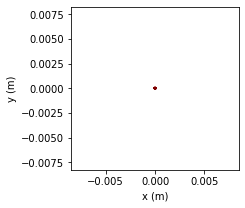The autoscale default from matplotlib does not always work and this is a good example. The distances, like the orbit of an electron inside a field of 1 T, are too small. We need to write an even better plotting function than plot_2D. This function is called

def plot_2D_autoscale(x,y,n_interpolated=1,plot_size=3,plot_axes="none"):


Note that this function has three optional arguments. n_interpolated corresponds to the number of interpolation points between time steps, as in plot_2D. It's default value is set to 1, i.e. no interpolation. plot_size sets the plot size to 3 by default. Finally, plot_axes is set to "none"

In :
def plot_2D_autoscale(x,y,n_interpolated=1,plot_size=3,plot_axes="none"):
n = len(x)
k = n_interpolated
#interpolation
x_interpolated = np.interp(np.arange(n * k), np.arange(n) * k, x)
y_interpolated = np.interp(np.arange(n * k), np.arange(n) * k, y)
#generate figure and axes
fig, ax = plt.subplots(1, 1, figsize=(plot_size, plot_size))
ax.scatter(x_interpolated, y_interpolated, c=range(n*k), linewidths=0,
marker='o', s=2*plot_size, cmap=plt.cm.jet,) #s is the size of the plotted symbol 'o'
ax.axis('equal')
#compute autoscale parameters
xc=(x.max()+x.min())/2.
x_low=xc-(x.max()-x.min())/2.*1.1
x_high=xc+(x.max()-x.min())/2.*1.1
yc=(y.max()+y.min())/2.
y_low=yc-(y.max()-y.min())/2.*1.1
y_high=yc+(y.max()-y.min())/2.*1.1
#set autoscale parameters
ax.set_xlim(x_low,x_high)
ax.set_ylim(y_low,y_high)
if (plot_axes!="axes"):#so we can switch the axis on or off
ax.set_axis_off()
else:
ax.set_xlabel("x (m)")
ax.set_ylabel("y (m)")

In :
plot_2D_autoscale(r[0,:],r[1,:],plot_axes="axes")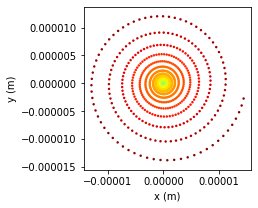Clearly, this is not a circle. Using the same strategy to solve this ODE did not work well here, as we are trying to approximate a second order ODE using a first order approach... Before, the underlying structure of motion was close to a straight line (hence first order). Now we have circular motion, which is second order, and cannot be approximated by a straight line. Here again we need to develop a model that preserves the underlying nature of motion. Doing a second order integration scheme is possible with python.

However, rather than using this method we are going to use something quite exotic called exponential integrators....

First, we have to notice that $$\vec F=q\vec v\times \vec B$$ can be rewritten as $$\frac{d\vec p}{dt}=\frac{q}{m}\vec p\times \vec B.$$ While this is not mathematically correct, we can see intuitively that $$\frac{d p}{dt}-\frac{q}{m}B p=0\tag{2.9}$$ This is a first order ordinary differential equation which has $$p=p_0e^{\frac{qB}{m}t}\tag{2.10}$$ as a solution. And so we think of using exponential integration. The physical model and the mathematical procedure to solve this equation is explained below. If you feel weary about the method, just glance over it.

According to the special law of relativity $$\frac{d\vec p^\mu}{dt}=\frac{q}{m\gamma}F_{\mu\nu}\vec p^\nu\tag{2.11}$$ where $F_{\mu\nu}$ is the contravariant electromagnetic field tensor given by $$F_{\mu\nu}= \begin{bmatrix} 0&E_x/c&E_y/c&E_z/c \\ -E_x/c&0&-B_z&B_y \\ -E_y/c&B_z&0&-B_x \\ -E_z/c&-B_y&B_x&0 \end{bmatrix}$$ and the contravariant four-momentum is $$\vec p^\nu= \gamma m \begin{bmatrix} c&v_x&v_y&v_z \end{bmatrix}.$$ $\gamma$ is the Lorentz factor which depends on the particle velocity as $$\gamma=\frac{1}{\sqrt{1-\frac{v^2}{c^2}}}$$ Eqs. $(2.7)$ and $(2.11)$ are equivalent when the particle velocity is small compare to the speed of light $c$.

Now, Eqs. $(2.9)$ and $(2.11)$ are really similar but for the fact that vectors have been omitted in Eq. $(2.9)$. It happens that the definition of the exponential function $$\exp(x) = \sum_{k = 0}^{\infty} {x^k \over k!} = 1 + {x \over 1!} + {x^2 \over 2!} + {x^3 \over 3!} + {x^4 \over 4!} + \cdots$$ can be extended to square matrices (since they multiply) as follow $$\exp(A) = \sum_{k = 0}^{\infty} {A^k \over k!} = 1 + {A\over 1!} + {A^2 \over 2!} + {A^3 \over 3!} + {A^4 \over 4!}.$$ We can show that the change in momentum with time simply follows from Eq. $(2.10)$ as $$\vec p ^\mu(t)=\exp\Big(\frac{q}{m\gamma}F_{\mu\nu}t\Big)\vec p ^\mu(0)$$ providing that $F_{\mu\nu}$ is constant in space and time. Note that $M=\exp\Big(\frac{q}{m\gamma}F_{\mu\nu}t\Big)$ is a matrix!

once we have computed the momentum, it can be integrated to obtain the trajectory. We can use a first order integration scheme to achieve this task $$\vec r_{t+\Delta t}=\vec v_{t+\Delta t}\Delta t+\vec r_t$$ However, we should use the fact that the solution is an exponential that can be integrated easily $$\vec r_{t+\Delta t}^\mu=\int_{t}^{t+\Delta t}\frac{1}{m\gamma}\exp(\frac{q}{m\gamma}F_{\mu\nu}s)ds\,\vec p^\mu_t+\vec r^\mu_t$$ While we can integrate this equation using linear algebra theory, we will integrate it numerically using the trapezoid rule.

We define Lorentz_factor(v) that computes the Lorentz factor as a function of a velocity $\vec v=(v_x,v_y,v_z)$

In :
def Lorentz_factor(v):
v2=norm(v)**2
c=299792458
return 1./sqrt(1-v2/c**2)


Now let's define two functions that describe the electric and magnetic field as a function of space and time. Beware that we will redefine these functions later in the notebook. So if you come back to this section these ones will be overwritten.

In :
def get_E(r,t):
return np.array([0,0,0])
def get_B(r,t):
return np.array([0,0,1])


For now, these functions hold constant fields. We will redefine them later with fields that are more complex.

Let's add another function that computes the electromagnetic field tensor $F_{\mu\nu}$

In :
def EM_field_tensor(E,B):
c=299792458
F_mu_nu=np.array([0,E/c,E/c,E/c,E/c,0,-B,B,E/c,B,0,-B,E/c,-B,B,0])
F_mu_nu.shape=(4,4)
return F_mu_nu


Let's now add two new functions and a library to measure the computational time

In :
from scipy.linalg import expm
from numpy.linalg import norm
import time


Let's set the initial conditions to study the trajectory of the electron inside a constant magnetic field.

In :
m=9e-31
q=-1.6e-19
c=299792458


It is now time to write our ODE integration using exponential integrators where we use the trapezoid rule to compute the trajectory from the momentum.

In :
def ODE_integration_exponential_integrator(initial_position,initial_velocity,time_frame,n_steps,n_integrate):
start_time = time.time()
loc=np.zeros((3,n_steps)) #3 for x,y,z
loc[:,0]=initial_position
v=initial_velocity
gamma=Lorentz_factor(v)
p_old=np.array([m*c,m*v,m*v,m*v])*gamma
p_new=np.zeros(4)
t=0
dt=(time_frame-time_frame)/n_steps
ds=dt/n_integrate
for i in range(1,n_steps,1): #solution of the momentum equation
B=get_B(loc[:,i-1],t)
E=get_E(loc[:,i-1],t)
F_mu_nu=EM_field_tensor(E,B)
p_new=np.matmul(expm(q/(m*gamma)*F_mu_nu*dt),p_old)
gamma=p_new/(m*c)
P=np.zeros((4,4))
s=0.
dPds=np.eye(4)*ds/2
x=np.zeros(4)
for j in range(0,n_integrate,1): #trapezoidal integration to get the trajectory
s+=ds
if (i>0):
P+=dPds
dPds=expm(q/(m*gamma)*F_mu_nu*s)*ds/2
if (i==0):
P+=dPds
P+=dPds
x=np.matmul(P,p_old)/(m*gamma)
loc[:,i]=x[1:4]+loc[:,i-1] #new position
p_old=p_new
t+=dt
#print("--- %s seconds ---" % (time.time() - start_time))
return loc


Can we make the function simpler? Let's compute the trajectory from the momentum using a simple first order integration scheme.

In :
def ODE_integration_first_order_exponential_integrator(initial_position,initial_velocity,time_frame,n_steps):
start_time = time.time()
loc=np.zeros((3,n_steps)) #3 for x,y,z
loc[:,0]=initial_position
v_old=initial_velocity
p_old=np.zeros(4)
p_old=m*c
p_old[1:4]=m*v_old
p_old*=Lorentz_factor(initial_velocity)
p_new=np.zeros(4)
dt=(time_frame-time_frame)/n_steps
f=np.zeros(3)
v_new=np.zeros(3)
gamma=Lorentz_factor(v_old)
t=0
for i in range(1,n_steps,1):
B=get_B(loc[:,i-1],t)
E=get_E(loc[:,i-1],t)
F_mu_nu=EM_field_tensor(E,B)
p_new=np.matmul(expm(q/(m*gamma)*F_mu_nu*dt),p_old)
gamma=p_new/(m*c)
v_new=p_new[1:4]/(m*gamma)
loc[:,i]=v_new*dt+loc[:,i-1] #new position
p_old=p_new
t+=dt
#print("--- %s seconds ---" % (time.time() - start_time))
return loc


Now let's run both functions and compare their results to the analytical solution of the Larmor radius given by Eq. $(2.8)$. We start with the one where the trapezoid rul i used to compute the trajectory from the momentum.

In :
r_initial=np.array([0,0,0])
v_initial=np.array([1000,0,0])
t_gyration=m/(abs(q)*norm(get_B(r_initial,0)))*(2*math.pi)
time_frame=[0,t_gyration]
print('Gyration time =',t_gyration,'s')
r=ODE_integration_exponential_integrator(r_initial,v_initial,time_frame,150,6)
print(r[0,:].min(),r[0,:].max())
plot_2D_autoscale(r[0,:],r[1,:],n_interpolated=1,plot_size=5,plot_axes="axes")

Gyration time = 3.534291735288518e-11 s
-5.6237435032659354e-09 5.623743503263859e-09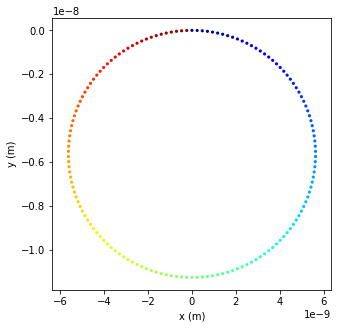This is a nice circle. And look at the value of the Larmor radius as computed analytically and and the one computed by the function. It's very accurate.

Now let's look at the other function. The one using the first order time integration for the trajectory.

In :
r=ODE_integration_first_order_exponential_integrator(r_initial,v_initial,time_frame,150)
print(r[0,:].min(),r[0,:].max())
plot_2D_autoscale(r[0,:],r[1,:],n_interpolated=1,plot_size=5,plot_axes="axes")

Larmor radius = 5.6250000000000016e-09 m
-5.743220979103771e-09 5.507601530084524e-09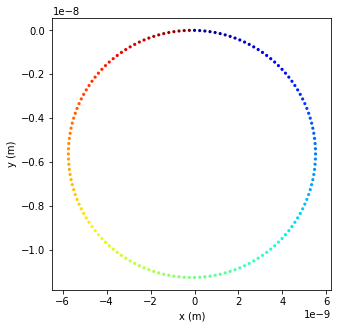All is good. The output looks like a circle. But pay attention to the value of the Larmor radius, as computed by the function and compare it to the analytical value. They are slightly different. Is this a problem?

Let's find out.

In :
time_frame=[0,109*t_gyration]
r=ODE_integration_first_order_exponential_integrator(r_initial,v_initial,time_frame,150)
print(r[0,:].min(),r[0,:].max())
plot_2D_autoscale(r[0,:],r[1,:],n_interpolated=1,plot_size=5,plot_axes="axes")

Larmor radius = 5.6250000000000016e-09 m
-2.980472608078362e-08 4.122206137687232e-09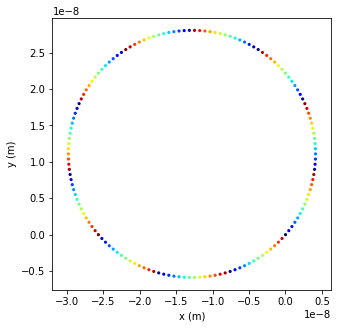The Larmor radius is completely off. The color are all jumbled up but this is due to aliasing! It is normal. Nothing to worry about.

In :
r=ODE_integration_exponential_integrator(r_initial,v_initial,time_frame,150,6)
print(r[0,:].min(),r[0,:].max())
plot_2D_autoscale(r[0,:],r[1,:],n_interpolated=1,plot_size=5,plot_axes="axes")

Larmor radius = 5.6250000000000016e-09 m
-5.349733178899195e-09 5.349733178685792e-09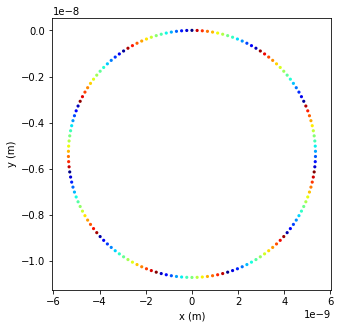Much better. After 109 gyration, we have roughtly the same error than ODE_integration_first_order_exponential_integrator had for one turn! You can check that both functions are actually giving good results, when the time step is small enough. However, you will discover that ODE_integration_exponential_integrator does much better for larger time steps, especially if you are increasing n_integrate. So, again, beware of time stepping! In fact, for inhomegeneous fields, this is n_steps that has to be increased.

## Particle trajectories inside homogeneous steady-state electric and magnetic fields¶

### The effect of parallel electric fields¶

Now we combine the effect of both electric fields and magnetic fields. If a magnetic field is given by $\vec B=(B_x,B_y,B_z)$, then the particle will gyrate in the $(x,y)$ plane. But what happens if there is also and electric field along the z-axis? Note that by parallel electric fields we mean parallel to $\vec B$. In this case, we need to solve the following system of differentiual equations. $$\frac{d^2v_x}{dt^2}=\frac{q}{m}\frac{dv_y}{dt}B_z\\ \frac{d^2v_y}{dt^2}=-\frac{q}{m}\frac{dv_x}{dt}B_z\\ \frac{dv_z}{dt}=\frac{q}{m}E_z$$ As before, we have a circular trajectory in the $(x,y)$ plane and a "free" fall along the $z$ axis.

Since we expect motion in all three directions, we need to write a new function that plots in three dimensions.

In :
def plot_3D_autoscale(loc,n_interpolated=1,plot_size=3,plot_axes="none"):
from mpl_toolkits.mplot3d import Axes3D
n = len(loc)
k = n_interpolated
#interpolation
x_interpolated = np.interp(np.arange(n * k), np.arange(n) * k, loc[0,:])
y_interpolated = np.interp(np.arange(n * k), np.arange(n) * k, loc[1,:])
z_interpolated = np.interp(np.arange(n * k), np.arange(n) * k, loc[2,:])
#generate figure and axes
fig = plt.figure(figsize=(plot_size,plot_size))
ax.scatter(x_interpolated, y_interpolated, z_interpolated, c=range(n*k), linewidths=0,
marker='o', s=2*plot_size, cmap=plt.cm.jet,) #s is the size of the plotted symbol 'o'
#compute autoscale parameters
xc=(loc[0,:].max()+loc[0,:].min())/2.
x_low=xc-(loc[0,:].max()-loc[0,:].min())/2.*1.1-1e-12
x_high=xc+(loc[0,:].max()-loc[0,:].min())/2.*1.1+1e-12
yc=(loc[1,:].max()+loc[1,:].min())/2.
y_low=yc-(loc[1,:].max()-loc[1,:].min())/2.*1.1-1e-12
y_high=yc+(loc[1,:].max()-loc[1,:].min())/2.*1.1+1e-12
zc=(loc[2,:].max()+loc[2,:].min())/2.
z_low=zc-(loc[2,:].max()-loc[2,:].min())/2.*1.1-1e-12
z_high=zc+(loc[2,:].max()-loc[2,:].min())/2.*1.1+1e-12
#set autoscale parameters
ax.set_xlim(min(x_low,y_low,z_low),max(x_high,y_high,z_high))
ax.set_ylim(min(x_low,y_low,z_low),max(x_high,y_high,z_high))
ax.set_zlim(min(x_low,y_low,z_low),max(x_high,y_high,z_high))
if (plot_axes!="axes"):#so we can switch the axis on or off
ax.set_axis_off()
else:
ax.set_xlabel("x (m)")
ax.set_ylabel("y (m)")
ax.set_zlabel("z (m)")

In :
def get_E(r,t):
return np.array([0,300,10])
def get_B(r,t):
return np.array([0,0,-1])
r_initial=np.array([0,0,0])
v_initial=np.array([1000,0,0])
t_gyration=m/(abs(q)*norm(get_B(r_initial,time_frame)))*(2*math.pi)
time_frame=[0,3*t_gyration]
r=ODE_integration_exponential_integrator(r_initial,v_initial,time_frame,150,6)
plot_3D_autoscale(r,n_interpolated=10,plot_size=8,plot_axes="axes")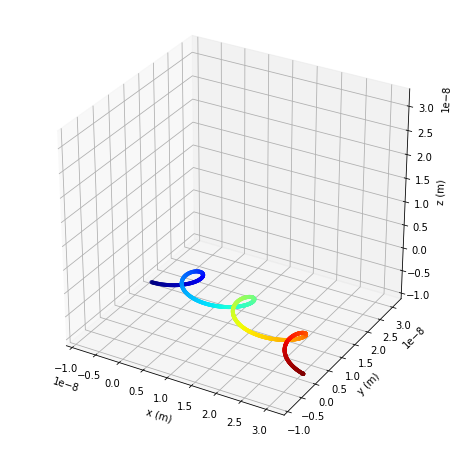We need to install a 3D plotting library now. It is called plotly. Open the terminal window from Anaconda Navigator and type conda install -c conda-forge plotly. It is also good for interactive 2D plots.

In :
def plotly_3D(loc,n_interpolated=1,sphere_size=1,opacity_value=0,scale_axis='x',autoscale=False):
import plotly.offline as py
import plotly.graph_objs as go
py.init_notebook_mode()
n = len(loc)
k = n_interpolated
#interpolation
x_interpolated = np.interp(np.arange(n * k), np.arange(n) * k, loc[0,:])
y_interpolated = np.interp(np.arange(n * k), np.arange(n) * k, loc[1,:])
z_interpolated = np.interp(np.arange(n * k), np.arange(n) * k, loc[2,:])
trace = go.Scatter3d(
x = x_interpolated,
y = y_interpolated,
z = z_interpolated,
mode='markers',
name = 'test',
marker=dict(
size=sphere_size,
color=np.linspace(0, 1, len(r[0,:])*k), # set color to an array/list of desired values
colorscale='Jet',   # choose a colorscale
opacity=opacity_value
)
)
xc=(loc[0,:].max()+loc[0,:].min())/2.
x_low=xc-(loc[0,:].max()-loc[0,:].min())/2.*1.1-1e-12
x_high=xc+(loc[0,:].max()-loc[0,:].min())/2.*1.1+1e-12
yc=(loc[1,:].max()+loc[1,:].min())/2.
y_low=yc-(loc[1,:].max()-loc[1,:].min())/2.*1.1-1e-12
y_high=yc+(loc[1,:].max()-loc[1,:].min())/2.*1.1+1e-12
zc=(loc[2,:].max()+loc[2,:].min())/2.
z_low=zc-(loc[2,:].max()-loc[2,:].min())/2.*1.1-1e-12
z_high=zc+(loc[2,:].max()-loc[2,:].min())/2.*1.1+1e-12
ratio_x=1
ratio_y=1
ratio_z=1
if (autoscale==False):
max_length=max(abs(x_high-x_low),abs(y_high-y_low),abs(z_high-z_low))
ratio_x=abs(x_high-x_low)/max_length
ratio_y=abs(y_high-y_low)/max_length
ratio_z=abs(z_high-z_low)/max_length
max_ratio=max(ratio_x,ratio_y,ratio_z)
ratio_x/=max_ratio
ratio_y/=max_ratio
ratio_z/=max_ratio

data = [trace]
layout = go.Layout(
autosize=False,
margin=dict(
l=0,
r=0,
b=0,
t=0
),
paper_bgcolor = 'grey',
scene = dict(
aspectratio = dict( x=ratio_x, y=ratio_y, z=ratio_z),
xaxis = dict(
gridcolor='rgb(255, 255, 255)',
zerolinecolor='rgb(255, 255, 255)',
showbackground=False,
backgroundcolor='rgb(230, 230,230)',
nticks=4, range = [x_low,x_high],),
yaxis = dict(
gridcolor='rgb(255, 255, 255)',
zerolinecolor='rgb(255, 255, 255)',
showbackground=False,
backgroundcolor='rgb(230, 230,230)',
nticks=4, range = [y_low,y_high],),
zaxis = dict(
gridcolor='rgb(255, 255, 255)',
zerolinecolor='rgb(255, 255, 255)',
showbackground=False,
backgroundcolor='rgb(230, 230,230)',
nticks=4, range = [z_low,z_high],),),
height =300,
width=500,
hovermode=False
)
fig = go.Figure(data=data, layout=layout)
py.iplot(fig, filename='3d-scatter-colorscale')

In :
#plotly_3D(r,n_interpolated=10,sphere_size=2,autoscale=False)


### EXB drifts¶

Let's suppose that we align the coordinate system along $\vec{B}$ so that $\vec{B}=B\hat z$ and $\vec E=E\vec x$, we have $$\frac{dv_x}{dt}=\frac{q}{m}(E+v_yB)\\ \frac{dv_y}{dt}=-\frac{q}{m}v_xB\\ \frac{dv_z}{dt}=0$$ If we have no initial velocity along the z-axis, then the trajectory is fully contained n the $(x,y)$ plane. Taking the derivative with respect to time and combining equations we find that $$\frac{d^2v_x}{dt^2}+\Big(\frac{qB}{m}\Big)^2v_x=0\\ \frac{d^2v_y}{dt^2}+\Big(\frac{qB}{m}\Big)^2v_y=-\Big(\frac{q}{m}\Big)^2BE$$ which solution is $$v_x=v\ cos(\omega t+\phi)\\ v_y=v\ sin(\omega t+\phi)-\frac{E}{B}$$ We have supposed that B and E are perpendicular here. To get the general expression of the drift we just have to take the component of $\vec E$ that is perpandicular to $\vec B$ or $$\vec v_{E\times B}=\frac{\vec E\times \vec B}{B}$$ Note that both positive and negative particles drift in the same direction.

In :
def get_E(r,t):
return np.array([0,10,0])
def get_B(r,t):
return np.array([0,0,1])
r_initial=np.array([0,0,0])
v_initial=np.array([10,0,0])
t_gyration=m/(abs(q)*norm(get_B(r_initial,time_frame)))*(2*math.pi)
time_frame=[0,11*t_gyration]
r=ODE_integration_exponential_integrator(r_initial,v_initial,time_frame,250,8)
#plotly_3D(r,n_interpolated=20,sphere_size=2,autoscale=False)


## Guiding center¶

When no physical processes take place on the cyclotron time scale, it is possible to simplify the trajectory by focusing only on the motion of the guiding center of the particle. The position of the guiding center is given by $$\vec r_p=\frac{1}{t_{cyclotron}}\int_0^{t_{cyclotron}}\vec r_p(t)dt$$ In this case, the particle motion is by adding all the velocities together:

1. Drifts relative to $\vec E$ $$\vec v_E = \left( 1 + \frac{1}{4}r_{Larmor}^2\nabla^2 \right) \frac{\vec E\times\vec B}{B^2}\tag{2.12}$$
2. Drifts relative to $\vec B$ $$\vec v_B =\frac{2eT}{qB}\frac{\vec{R}_c\times\vec{B}}{R_c^2 B}\tag{2.13}$$

In Eq. $(2.13)$ the temperature $T$ is in $V$ and $e$ is the elementary charge. The term $eT$ is in joules. It correspond to the microscopic energy carried by particles in a Maxwellian distribution. Note that to convert $volt$ to $kelvin$ one can use the fact that $k_BT(K)=eT(V)$. Eq. $(2.13)$ also has the radius of curvature $\vec R_c$ of the particle.

This representation is relatively limited in scope. It breaks down when large gradients or fast time variations occur as the particle moves along its trajectory. However, computations based on Eqs. $(2.12)$ and $(2.13)$ are faster than using Eq. $(2.11)$

## Problems¶

### Problem 1¶

Compare the final positions of the particle as computed by the numerical integration of the ordinary differential equation using ODE_integration_E and compare it to the analytical (i.e. correct) integration given by Eq. $(2.5)$. To do this, plot the final distance obtained by ODE_integration_E along the y-axis as a function of the time step and show that it converges towards the value of the analytical solution

### Problem 2¶

Compute the angle of deflection of an electron "flying by" a fixed ion as a function of the initial velocity of the electron. Infer the dependence of the angle of deflection with velocity. Comment on how the discretization impacts the angle at low velocities.

### Problem 3¶

Plot the strength (color) and direction (arrows) of the electric field produced by one single charge in 2D. Try to do it in 3D after that. While it is not that difficult, 3D plot tend to be difficult to read if not done properly.

### Problem 4¶

1. Write a piece of code to compare the deviation between ODE_integration_exponential_integrator and the actual Larmor radius. We call the difference between them the error $e$.
2. Vary the number of time steps and the number of integration steps and plot how the error $e$ varies as a function of both.
3. What can you conclude?

### Problem 5¶

When electric fields and magnetic fields are presents, charged particles do not simply gyrate, they move along the electric field direction also. When the electric field is purely perpendicular to B, the charge particle is said to drift, hence the name ExB drift. We are going to use ODE_integration_exponential_integrator to compute the drift velocity of an electron inside a magnetic field $\vec B=(0,0,1)$ and in an electric field $\vec E=(100,0,0)$.

1. Compute analytically the gyration period $t_{Larmor}$
2. What is its numerical value?
3. Starting with $t_{initial}=0$ and ending at $t_{final}=5\,t_{Larmor}$, find the distance traveled by the electron and infer the velocity from this value.
4. Redo 1,2 and 3 for a proton. Is the proton traveling in the same direction?

### Problem 6¶

If you remember we had a little problem with OED_integration_E. If we integrated our ODE for too long, then an electron inside a 1V electric field would eventually go faster than the speed of light. ODE_integration_exponential_integrator takes into account relativistic correction and really avoid this problem.

1. Using the exact same initial values of position and velocity, find out what is the velocity of the electron after 3s. If it does go faster than the speed of light, increase the number of time steps.

Let's imagine that you want to build a particle accelerator using a steady electric field of $1kV/m$ to accelerate an electron to $0.999c_{light}$

1. How long should the accelerator be?
2. How long should it be if you ignore relativistic corrections, i.e. use OED_integration_E?

© Pierre Gourdain, The University of Rochester, 2020

In [ ]: